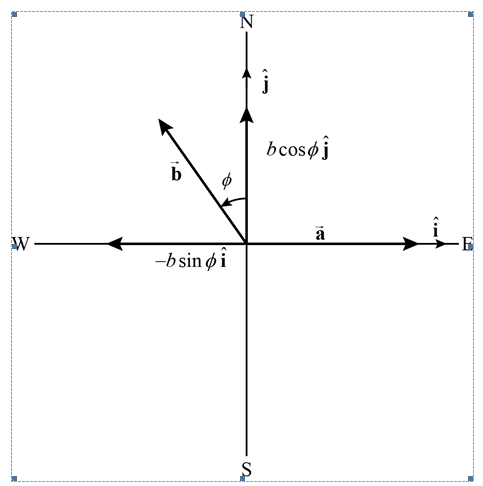Click to Chat

1800-1023-196

+91-120-4616500

CART 0

• 0

MY CART (5)

Use Coupon: CART20 and get 20% off on all online Study Material

ITEM
DETAILS
MRP
DISCOUNT
FINAL PRICE
Total Price: Rs.

There are no items in this cart.
Continue Shopping
        	vector a has  Magnitude of 5.2 units and is directed east .vector b has a magnitude of 4.3 units and is directed 350 of north .By construtig vector diagam. find the magnitude and direction of (a+b)and (b)a-b.
one year ago

							Therefore the vector is . Substituting the above values in equation (3), we haveFrom equations (1) and (2), we have , we have .along the unit vector …… (3)Since there is no component of vector is given as: and vector that is along west.(a) The addition of vector indicates that the component points opposite to the direction of unit vector of vector …… (2)The negative sign in the componentSubstituting the given values, we have …… (1)The vector is given as: is equal to the magnitude of vector that is a .Substituting the given values, we have, the value of componentpoints in the east, along the unit vector  .Since vector  along the unit vectorWhere is the component of vector as:Therefore one can write the vectorGiven:(refer figure below).is  is given as whereas the vector component along the west, opposite to the direction of unit vector  towards the north, along the unit vector  is represented by a .Therefore the vector component of vector be represented by b whereas the magnitude of vectordegrees west of north. We also assume that the magnitude of vector is such that vector is points toward the east direction (as can be seen from the figure above).We assume that the angle subtended by vectorpoints towards the north while the unit vector respectively. The unit vector  and into its components along the unit vectorsTo add the vectors, we need to resolve vector. vectors and The figure below shows the vector diagram, representing

one year ago
Think You Can Provide A Better Answer ?

## Other Related Questions on Mechanics

View all Questions »### Course Features

• 101 Video Lectures
• Revision Notes
• Previous Year Papers
• Mind Map
• Study Planner
• NCERT Solutions
• Discussion Forum
• Test paper with Video Solution### Course Features

• 110 Video Lectures
• Revision Notes
• Test paper with Video Solution
• Mind Map
• Study Planner
• NCERT Solutions
• Discussion Forum
• Previous Year Exam Questions